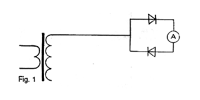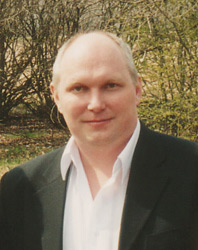The Single-Wire Electric Power Transmission
By Alexander V. Frolov

Any motion of charges is electric current by definition. The electric potential field can also move the electric charges and this work of the potential field is not connected with loss of power. So, it is enough to use the electrical field (scalar potential source) to create the power and work in an electric load circuit.

The classic conception does not explain this paradox but states: 'The total work of the potential field along a closed trajectory is equal to zero." That is correct, sure. But there are simple descriptions of
experiments for application of potential field energy to create power in a load . The present paper
develops this concept from another view.

So, the motion of charged particles is the current. But there are both the wattful current and wattless
current. To create the free energy system it is necessary to transform the wattless current to wattful
current.

What is the difference in those two versions of current? When the charged particles are moving along wire thanks to electromotive force of potential difference, it is not the reason for loss of power in the source because the electric field of the primary source provides the work to move charged particles without any power loss. A closed electrical circuit is the reason to consume the potential difference of the source. It is possible to separate the load current from source circuit. Dr. T.E. Bearden made detailed description of a concept for this technology by means of note for "the
massless current" . Massless current is the wattless current, from my point of view.

It is possible to consider such current as oscillations of a field of free electrical charges (3]. In this case the wattless current is described as displacement current or as longitudinal wave of the electrical field.

Let's consider a simple experiment to prove the possibility of power transmission by means of
displacement current. The equipment that I used:
1) Generator, output voltage is 30 Volts, frequency is 10 KHz or more.
2) Electromagnetic transformer to increase the output voltage from 30 Volts to several kiloVolts (2-5 kV in my scheme).
3) Diodes are connected as shown on Figure 1. This diodes connection is so-called "Avramenko's plug" .Fig. 1
There is a high voltage version of this experiment and a high frequency version. For the first version, the load on "Avramenko's plug" is an electrical condenser of 10 nanoFarad capacity for maximum voltage 3 kV, for example. The frequency is from 5 to 10 KHz. Both  legs of condenser are bent to make a spark discharger.

I used a spark distance from 0.5 to 1 mm. The frequency of spark discharges in my experiment varied from 1 Hz to 10 Hz. It is possible to calculate the work  for one discharge by means of next formula:

A = 0.5 CV2

For power we can use the formula:

P=A/T=Af

where f is frequency of spark discharges.

So, for this simple "home laboratory" experiment when the voltage V = 2 KV, capacity C =10 nanoFarad, and f = 5 Hz, the P is equal to 0.1 watt. The consumed power is equal to 3 watts DC (0.1 A and 30 V). Therefore, power in a closed circuit of load is about 3% of consumed power in this experiment. But this small power is free since it is not connected with conduction current in source circuit. There is only wattless displacement current in single-wire part of the system.

For high frequency version it s possible to use the voltage near 30 V and the frequency of generator more than 100 KHz. Simple electric lamp can be used as load of the "Avramenko's plug" in this version. By Avramenko, the conduction current is load is 60 mA when voltage V =50 Volts and frequency of generator is 100 KHz . So, load power in Avramenko's experiments is equal to 3 Watts.

From my experiments, it is possible to make some  conclusions:
1. When the output signal is a sine wave there is no difference for wires of secondary coil of transformer and any wire can be connected to diodes. In any case, the power in load is the same. But when the output signal is unipolar pulses (from output of transistor scheme, for example) there is important difference for wires of coil. When the diodes are connected to positive pulsed pole of coil the power in load is maximum. The same difference is easy to verify if one is to bring metal material to end of wires of high voltage coil of transformer. The discharge between metal and positive pulsed wire is more  powerful than discharge between metal and other wire of the coil.

This note can be explained in conception of longitudinal waves as waves of electron gas in matter.
When the positive potential take place on the wire the electrons of metal are attracted to positive potential.  The spark discharge between metal and wire takes place here since electrons of metal can "jump" from metal into positive charged end of coil wire. In opposite case, electrons of metal are in repulsion from negative charged end of the coil wire. There is no condition for electrons to "jump" in this case. So, positive pulses of potential field can lead to conduction current. In a metal piece, the "jumped   electrons" are compensated by electrons from air. In a closed circuit that uses the Avramenko's plug," electrons can be moving only in one direction and it is the reason for producing work in the load.

2. To increase the power in the load, it is necessary to develop that part of the scheme that is responsible for the displacement current. The output power of the generator is not important. Small power is enough to create the wattless current. The question of power in load is the question of amplitude. It is possible to create high values of amplitude for a longitudinal wave of displacement current in a resonance mode.

3. The principle, in general: the electric potential field is the cause for free wattless movement of charged particles (electrons or ions). This movement can be used for power generation. Most
interesting is the correlation of this experiment with  known electric induction phenomenon. The
development of such technologies is most favorable in this direction, I think.

Finally, I must note that N. Tesla demonstrated the transmission of power along a single wire in London in 1892 . Now all we need is the real interest of industry and official science to this well-known technology for clean power generation by means of potential source.

References

1. A.V. Frolov, "The Application of Potential Energy for Creation of Power, New Energy News, vol. 2, no 2. May 1994.
2. T. E. Bearden, "Overunity Electrical Power Efficiency Using Energy Shuttling Between Two Circuits,"  Proceedings of the 2nd international New Energy Symposium, Denver, Co, May 13-15. 1994.
3. "New Electric Transmission," summarized by Igor Goryachev, New Energy News, vol. 2, no 6, October 1994.
4. N.E. Zaev, S.V. Avramenko, V.N. Lisin, "The Measuring of Conduction Current That is Stimulated by Polarization Current," Journal of Russian Physical Society, no 2, 1991. Russian Text.